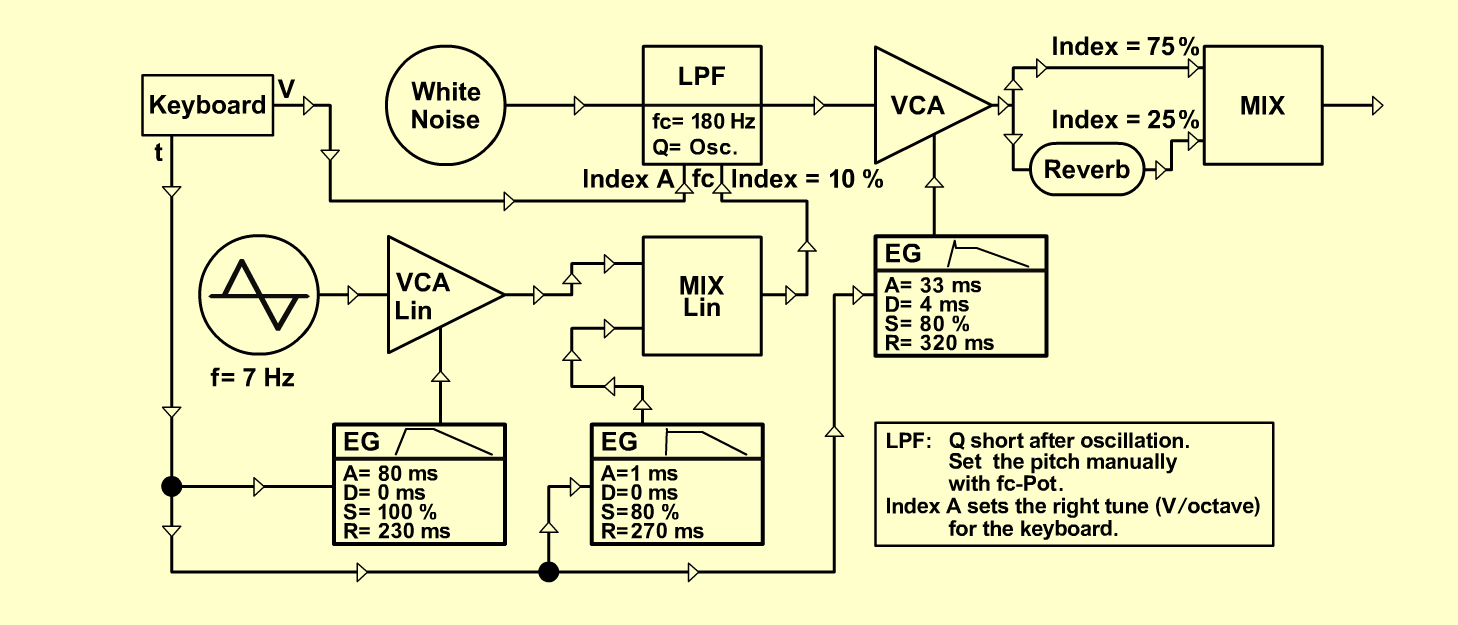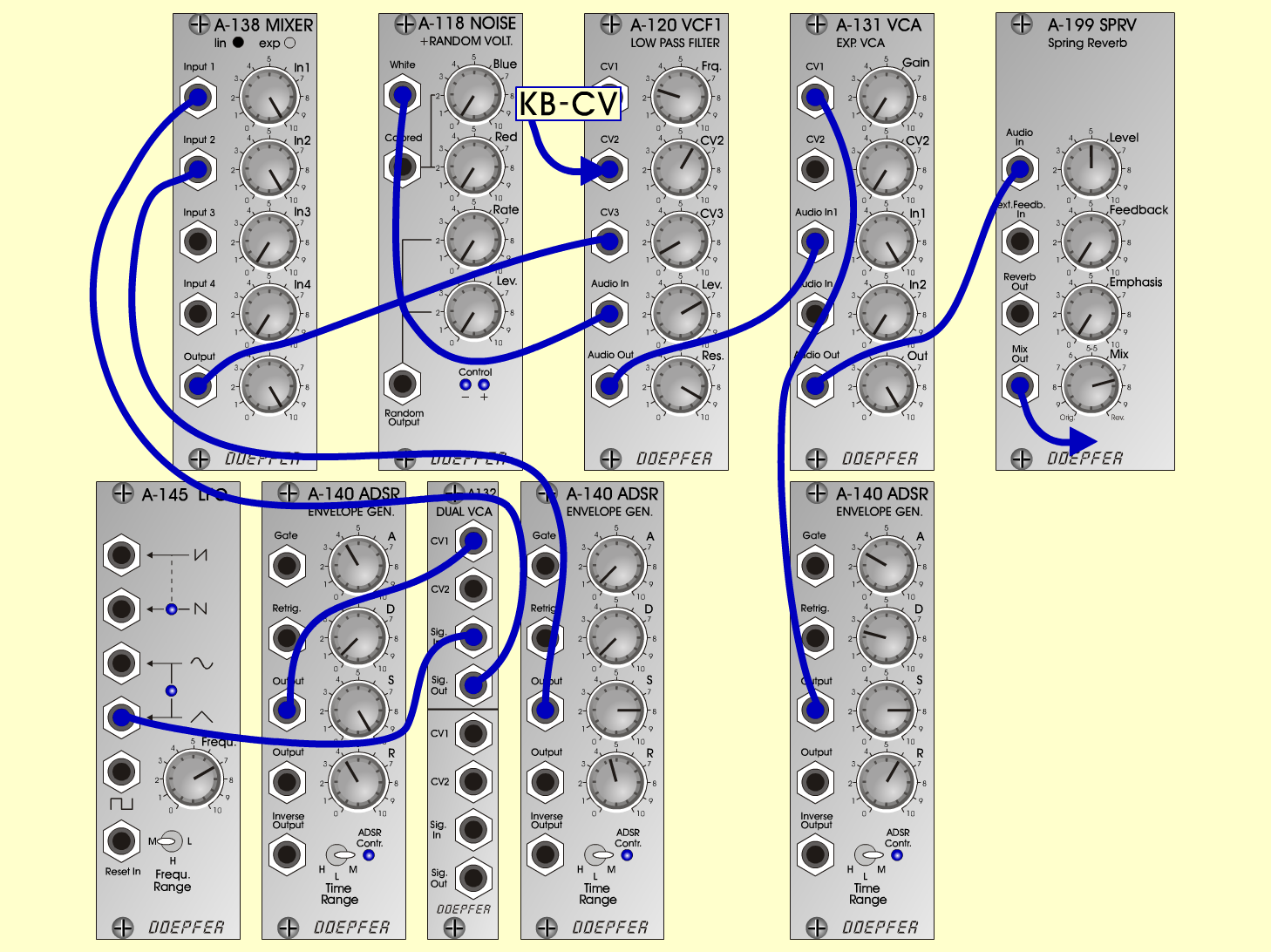WhistleInstrumentsD o e p f e r A - 100 Connections: Settings: A-118 (White)  <=>  A-120 (Audio In)   A-145 (Triangle) <=>  A-132 (Sig. In)   A-140/1 (Output) <=>  A-132 (Sig. In)   A-132 (Sig. Out)  <=>   A-138/lin (Input 1)   A-140/2 (Output) <=>   A-138/lin (Input 2)   A-138/lin (Out) <=>   A-120 (CV 3)   A-120 (Audio Out) <=>   A-131 (Audio In)   A-140/3 (Output) <=>   A-131 (CV 1)   A-131 (Out) <=>   A-199 (Audio In)   For trigger and gate:   KB (t, g)  <=> A-140 (Gate)   For pitch:   KB (V) <=>  A-120 (CV 2) A-145 (Frq = 7, Range = M)   A-138/lin (In 1 = 10, In 2 = 10, Out = 10)   A-140/1 (A = 4, D = 0.5, S = 10, R = 4,    Range = M)   A-140/2 (A = 0.5, D = 0.5, S = 8, R = 4.5,   Range = M)   A-140/3 (A = 3, D = 2.5, S = 8, R = 6,   Range = M)   A-120 (Freq = 2.6, CV2 = 6, CV3 = 1, Lev = 7,   Res = 9)   A-131 (Gain = 0, Audio In 1 = 10, Audio Out = 10)   A-199 (Lev= 5, Feedb = 0, Emph = 0,   Mix = 7.5 orig) Notes: A-120: Res short after oscillation.       Set  the pitch manually with Freq-Pot.       The CV 2-Pot sets the right tune (V /octave)       for the keyboard.       Use for fine tuning an A-176 (CVS). Josef MuellerSound samples Whistle Siciliano (Bach BWV 1031)     Second instrument: Pizzicato Whistle.mp3 (261 Hz) Whistle.mp3 (523 Hz)